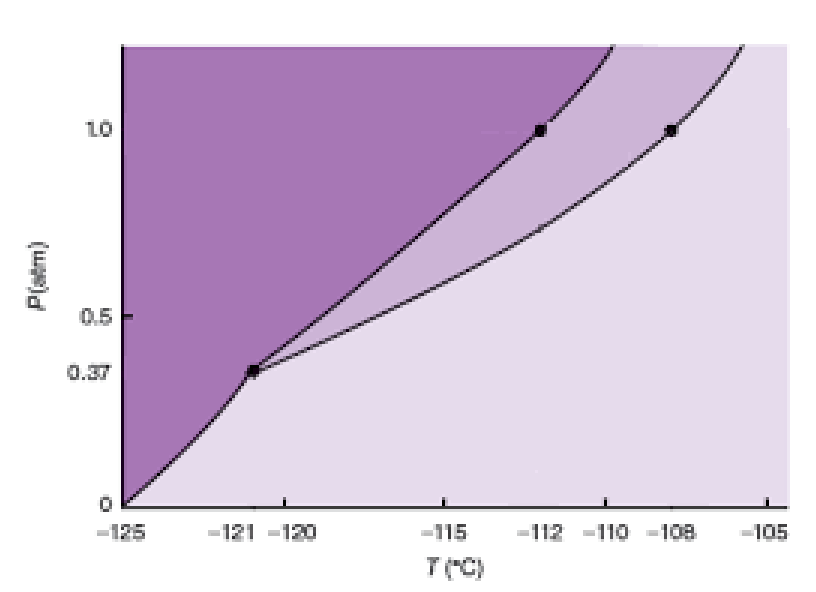# Problem: The phase diagram for xenon is shown below:What phase change occurs if the temperature at 0.35 atm drops from -118 to -124°C?

###### FREE Expert Solution

Analyze the given phase diagram and determine temperature when the equilibrium vapor pressure is at 380 mmHg

Recall that a phase diagram shows the transition of matter between solid, liquid, and gas phases as temperature and pressure changes.

In a phase diagram, the different phases can be identified by their location:

• Solid: can be found at high pressure and low temperature

• Liquid: can be found between the solid and gas regions

• Gas: can be found at low pressure and high temperature

82% (24 ratings)###### Problem Details

The phase diagram for xenon is shown below:What phase change occurs if the temperature at 0.35 atm drops from -118 to -124°C?